# Störmer method

A finite-difference method for finding a solution to the Cauchy problem for a system of second-order ordinary differential equations not containing the first derivative of the unknown function:Integrating over a grid with a constant step,gives the following computational formulas:

a) extrapolation: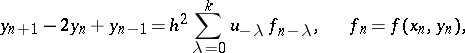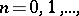or (in difference form)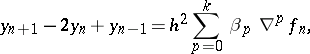where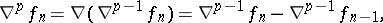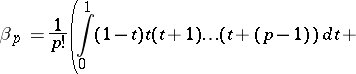b) interpolation: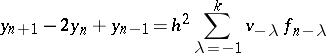or (in difference form)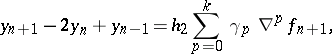where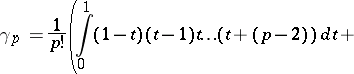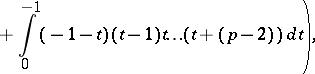The first values of the coefficients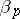and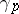are: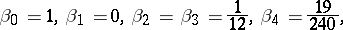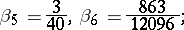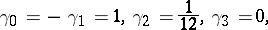For one and the same, formula b) is more accurate, but requires the solution of a non-linear system of equations to obtain the value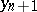. In practice, one first obtains an approximation for the solutionby formula a), and then makes it more precise by applying the formula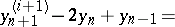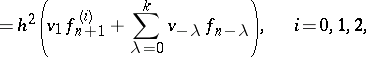Application of Störmer's method is based on the assumption that the approximate values of the solution at the firstpoints of the grid,(supporting values), are already known. These values are either computed by the Runge–Kutta method or by using the Taylor expansion of the solution. The necessity to apply special formulas to compute the values at the beginning of the process and when changing the step of the grid over which the integration is carried out leads to essentially more complicated computer programs.

Störmer's formulas withterms on the right-hand side have an error of order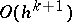. The estimate of the error is similar to the corresponding estimate for the Adams method. One can show that for anythere are stable formulas with an error of order.

In practice, one usually uses formulas with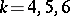. One of Störmer's interpolation methods, called the Numerov method, is widely used:The method was introduced by C. Störmer in 1920.